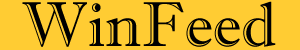The Cheapest Least Cost Feed Formulation Software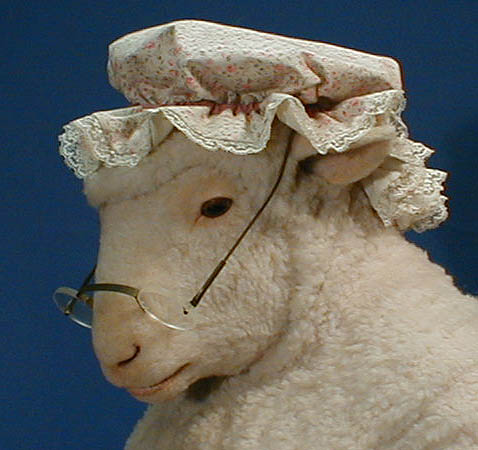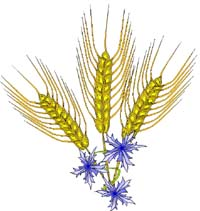Linear Feed Formulation Simple diets can be formulated using either Pearson’s Square or simultaneous equations methods. Both of these methods are unable to handle inequalities or ranges and both are independent of price. Conventionally "Least Cost Feed Formulation" is done using Linear Programming. This method was first developed in 1947 by G.B. Dantzig to solve some U.S. Air Force planning problems but now it is widely used in all types of fields. A standard linear programming model, in matrix form, may be stated as follows.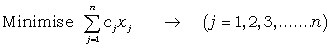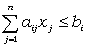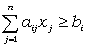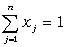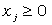where cj = Cost per unit for jth ingredient xj = Quantity of jth ingredient aij = Quantity of ith nutrient per unit of jth ingredient bi = Requirement for ith nutrient in the diet Almost all commercial feed formulation software use this method to formulate diets. In real life nutrient composition is highly variable. This variation is associated with variety of factors which include variation of nutrient content of ingredients coming from different batches and sources and variation attributed to the laboratory procedure and human error. For example if same sample of soybean is analyzed multiple times for protein content, it is very likely that every time a slightly different value will be obtained. In Linear Programming method a mean value of these analytical values is used for formulation. Statistically, these mean values are associated with only 50% confidence of meeting the requirements in prepared formula. An alternative solution to this problem is use of Stochastic Programming for feed formulation. WinFeed has capability of formulating diets by this method. To learn more about Stochastic Feed Formulation click here.